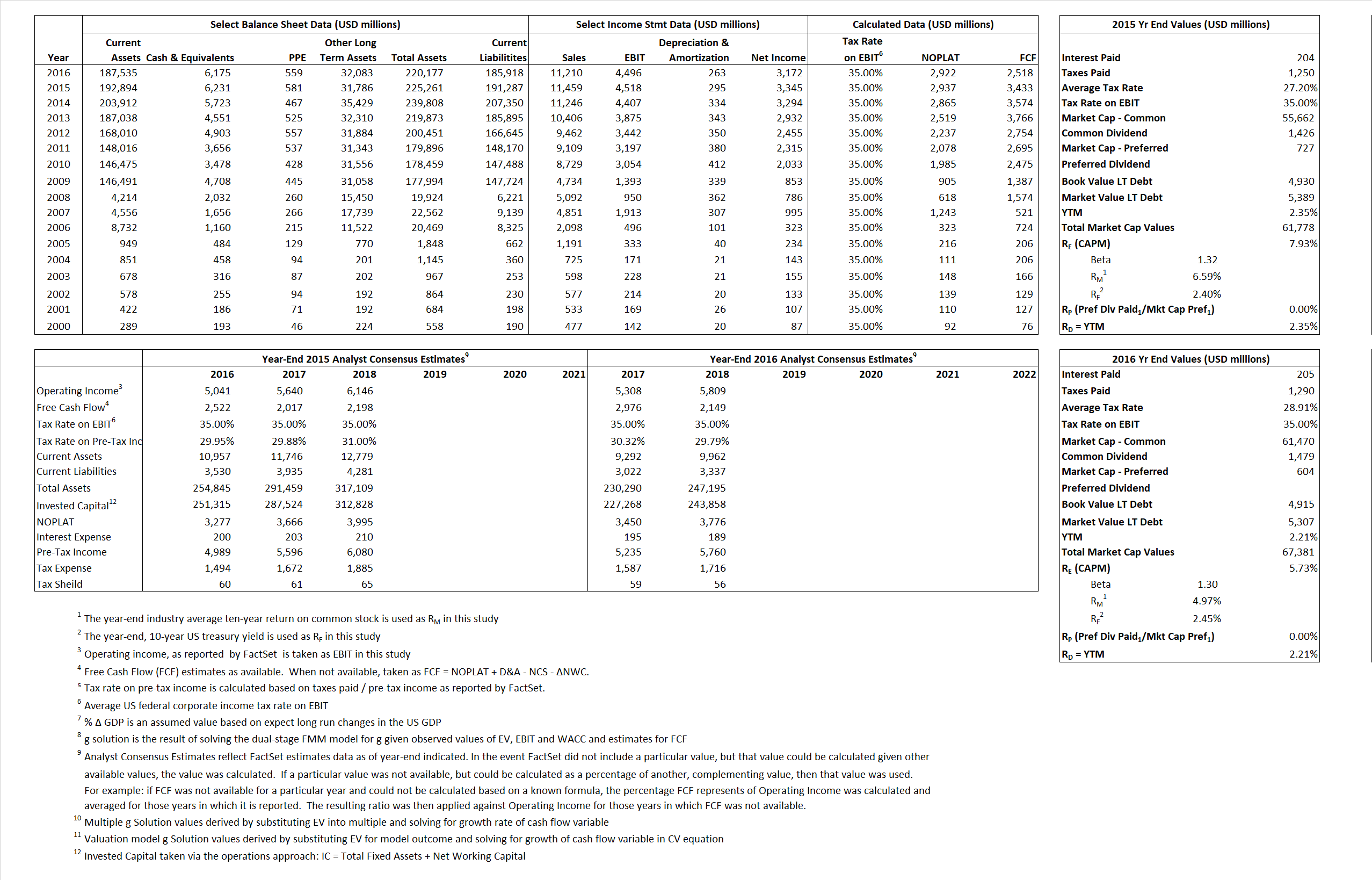# BlackRock Inc

## Analyst Listing

The following analysts provide coverage for the subject firm as of May 2016:

 Broker Analyst Analyst Email Deutsche Bank Research Brian Bedell brian.bedell@db.com Wells Fargo Securities Christopher Harris christopher.harris1@wellsfargo.com Credit Suisse Craig Siegenthaler craig.siegenthaler@credit-suisse.com Jefferies Daniel T. Fannon dfannon@jefferies.com RBC Capital Markets Eric N. Berg eric.berg@rbccm.com Evercore ISI Glenn Schorr glenn.schorr@evercoreisi.com Piper Jaffray Jason Weyeneth jason.l.weyeneth@pjc.com Bernstein Research Luke Montgomery lucas.montgomery@bernstein.com Gabelli & Company Macrae Sykes msykes@gabelli.com Keefe Bruyette & Woods Robert Lee rlee@kbw.com

## Primary Input Data## Derived Input Data

### Equational Form

Net Operating Profit Less Adjusted Taxes NOPLAT  2,937  2,922$NOPLAT\, =\, EBIT\, x\, (1 \,-\, Avg \,\,Tax\,\, Rate\,\, on\,\, EBIT)$
Free Cash Flow FCF 3,433 2,518$FCF\,=NOPLAT\,+\,Non-Cash\,Expenses-\Delta NWC\,-\,NCS$
Tax Shield TS 55 59$TS\,=\,Interest\,\,Paid\,\,x\,\, Avg \,\,Tax\,\,Rate\,\, on\,\, Pre-Tax\,\, Income$
Invested Capital IC 33,974 34,259$IC\,=\,Fixed\,\,Operating\,\,Assets\,\,+\,\,Net\,\, Working\,\, Capital$
Return on Invested Capital ROIC 8.64% 8.53%$ROIC\,=\,\frac { NOPLAT }{ IC }$
Net Investment NetInv 1,811 548$NetInv\,=\,{ {IC}_{1}}-{{IC}_{0}}+Depreciation$
Investment Rate IR 61.67% 18.75%$IR\,=\,\frac {NetInv}{NOPLAT}$
Weighted Average Cost of Capital
WACCMarket 7.29% 5.35%$WACC\,=\,\frac { E }{ V } { R }_{ E }\,+\,\frac { P }{ V } { R }_{ P }\,+\,\frac { D }{ V } { R }_{ D }\left( 1- Avg\,\, Tax\,\,Rate\,\,on\,\,Pre-Tax\,\,Income \right)$
WACCBook  10.93%  10.27%
Enterprise value
EVMarket  55,547  61,206$EV\,=\,Market\,\,Cap\,\,Equity\,+\,\,Long\,\,Term\,\,Debt\,-\,Cash$
EVBook  55,088  60,814
Long-Run Growth
g = IR x ROIC
5.33%  1.60% Long-run growth rates of the income variable are used in the Continuing Value portion of the valuation models.
g = %$\Delta$ GDP  2.50%  2.50%
Margin from Operations M 39.43%  40.11%$M\,\,=\,\,\frac{EBIT}{SALES}$
Depreciation/Amortization Rate D 6.13%  5.53%$D\,\,=\,\,\frac{D+A}{EBITDA}$

## Valuation Multiple Outcomes

The outcomes presented in this study are the result of original input data, derived data, and synthesized inputs.

### model g solution

12/31/2015 12/31/2016 12/31/2015 12/31/2016 12/31/2015 12/31/2016

EV/SALES$\frac {EV}{Sales} \,= \,\frac{ROIC\, -\, g}{ROIC\,(WACC\,-\,g)}\,(1\,-\,T)\,(M)$

4.85

5.46

5.17%  1.30%  5.62%  2.11%

EV/EBITDA$\frac {EV}{EBITDA} \,= \,\frac{ROIC\, -\, g}{ROIC\,(WACC\,-\,g)}\,(1\,-\,T)\,(1\,-\,D)$

11.54 12.86 5.17% 1.30% 5.62% 2.11%

EV/NOPLAT$\frac {EV}{NOPLAT} \,= \,\frac{ROIC\, -\, g}{ROIC\,(WACC\,-\,g)}$

18.91 20.94 5.17% 1.30% 5.62% 2.11%

EV/FCFOPS$\frac {EV}{FCF_{OPS}} \,= \,\frac{ROIC\, -\, g}{ROIC\,(WACC\,-\,g)}\,(1\,-\,T)$

16.18 24.31 5.17% 1.30% 5.62% 2.11%

EV/EBIT$\frac {EV}{EBIT} \,= \,\frac{ROIC\, -\, g}{ROIC\,(WACC\,-\,g)}\,(1\,-\,T)$

12.29 13.61 5.17% 1.30% 5.62% 2.11%

EV/IC$\frac {EV}{IC} \,= \,\frac{ROIC\, -\, g}{WACC\,-\,g}$

1.63 1.79 5.17% 1.30% 5.62% 2.11%